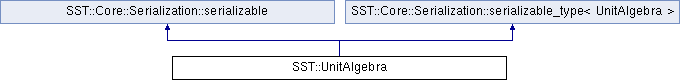SST  6.0.0 StructuralSimulationToolkit
SST::UnitAlgebra Class Reference

Performs Unit math in full precision. More...

`#include <unitAlgebra.h>`

Inheritance diagram for SST::UnitAlgebra:## Public Member Functions

UnitAlgebra (std::string val)
Create a new UnitAlgebra instance, and pre-populate with a parsed value. More...

void print (std::ostream &stream)
Print to an ostream the value.

void printWithBestSI (std::ostream &stream)
Print to an ostream the value Formats the number using SI-prefixes.

std::string toString () const
Return a string representation of this value.

std::string toStringBestSI () const
Return a string representation of this value Formats the number using SI-prefixes.

UnitAlgebraoperator= (const std::string &v)

UnitAlgebraoperator*= (const UnitAlgebra &v)
Multiply by an argument;.

template<typename T >
UnitAlgebraoperator*= (const T &v)
Multiply by an argument;.

UnitAlgebraoperator/= (const UnitAlgebra &v)
Divide by an argument;.

template<typename T >
UnitAlgebraoperator/= (const T &v)
Divide by an argument;.

bool operator> (const UnitAlgebra &v) const
Compare if this object is greater than the argument.

bool operator>= (const UnitAlgebra &v) const
Compare if this object is greater than, or equal to, the argument.

bool operator< (const UnitAlgebra &v) const
Compare if this object is less than the argument.

bool operator<= (const UnitAlgebra &v) const
Compare if this object is less than, or equal to, the argument.

UnitAlgebrainvert ()
Apply a reciprocal operation to the object.

bool hasUnits (std::string u) const
Returns true if the units in the parameter string are found in this object.

sst_dec_float getValue () const
Return the raw value.

int64_t getRoundedValue () const
Return the rounded value as a 64bit integer.

void serialize_order (SST::Core::Serialization::serializer &ser)Public Member Functions inherited from SST::Core::Serialization::serializable
virtual const char * cls_name () const =0

virtual uint32_t cls_id () const =0Protected Types inherited from SST::Core::Serialization::serializable
enum  cxn_flag_t { ConstructorFlag }Static Protected Member Functions inherited from SST::Core::Serialization::serializable
static void serializable_abort (uint32_t line, const char *file, const char *func, const char *obj)

## Detailed Description

Performs Unit math in full precision.

Allows operations such as multiplying a frequency by 2.

## Constructor & Destructor Documentation

 UnitAlgebra::UnitAlgebra ( std::string val )

Create a new UnitAlgebra instance, and pre-populate with a parsed value.

Parameters
 val Value to parse. It is of the following format: val := NUMBER( )?UNITS NUMBER := (-)?[0-9]+(.[0-9]+)? UNITS := UNITGROUP(/UNITGROUP) UNITGROUP := UNIT(-UNIT)* UNIT := (SIPREFIX)?(BASEUNIT|COMPUNIT) SIPREFIX := {a,f,p,n,u,m,[kKMGTPE]i?} BASEUNIT := {s,B,b,events} COMPUNIT := {Hz,hz,Bps,bps,event}

The documentation for this class was generated from the following files: